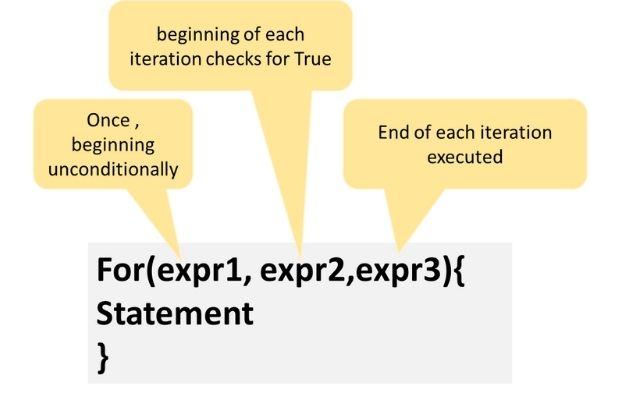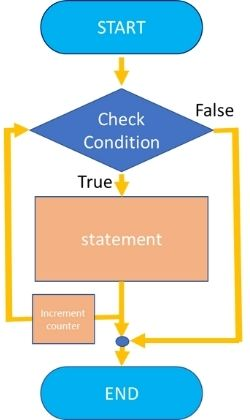# For & foreach looping

``````for(\$i=1; \$i<=10; \$i++){
echo \$i."<br>";
}``````
This will list from number one to ten.

In above case the loop is executed 10 times and each time the value of \$i is increased by 1. So in the first loop the value of \$i became 1 and in the last loop the value equals to 10. Next time when the value of \$i becomes 11 the condition checking fails and the loop is escaped without executing. Here condition is checked ( Expr 2) at the staring of the loop.This is used frequently in many scripts where a common block of code to be executed repeatedly. We can add break statement to stop the loop in between based on matching requirements.

For and foreach loop in PHP with break statement and creating patterns using nested for loops

Expr3 is executed at the end of the loop. Check the ouput just outside the for loop.
``````for(\$i=1; \$i<=5; \$i++){
echo \$i."<br>";
}
echo " Value of \\$i Outside the loop: ".\$i;``````
Output is here
``````1
2
3
4
5
Value of \$i Outside the loop: 6``````

## break;

By using break we can exit the loop without completing the total required loopings (early exit).
``````for(\$i=1; \$i<=10; \$i++){
if(\$i > 5){break;}
echo \$i."<br>";
}``````
This will list from 1 to 5 only. The If condition inside the for loop will exit the loop when the value of \$i exceeds 5 ( that is when it equals to 6)

## continue

continue is used within looping structures to skip the rest of the current loop iteration and continue execution at the condition evaluation and then the beginning of the next iteration.
``````for(\$i=1; \$i<=10; \$i++){
if(\$i == 5){continue;} // When value=5, below line is skiped.
echo \$i."<br>";
}``````
Above code will print 1 to 10 except the number 5.

## break and continue

``````for(\$i=1; \$i<=10; \$i++){
if(\$i == 5){continue;} // When value=5, below echo line is skiped.
if(\$i == 7){break;} // When value=7, the loop is skiped.
echo \$i."";
}``````
Output is 1,2,3,4 and 6.

## Step value of increment in loop

So far we have seen each time the variable value is increased by 1. This increase or step value can be changed. Now we will use a step value of 10 so the variable \$i will increase its value by 10 ( it was increasing by 1 in previous cases ) in each looping.
``````for(\$i=0;\$i<=100;\$i +=10){
echo \$i."<br>";
}``````
The above code will display 0,10,20 ... till 100

Noter that `\$i += 10;` is same as `\$i=\$i+10;`.
Similarly `\$i -=10;` is same as `\$i=\$i-10;`

## Decrement step value in loop

We can reduce the step value in each step of iteration.
``````for(\$i=10;\$i>0;\$i--){
echo \$i."<br>";
}``````

## foreach()

While handling PHP Arrays foreach function is required to display the elements of an array. Once the foreach function is called the array pointer will reset to the first element of the array. So we need not call the reset() again( Reset() rewinds array's internal pointer to the first element. ).
Here is an example of the foreach code.
``````\$a = array (10, 12, 13, 25);

foreach (\$a as \$i) {
print "Present  value of \\$a: \$i <br> ";
}``````
``````\$ar=array("FName"=>"John","Address"=>"Streen No 11 ",
"City"=>"Rain Town","Country"=>"USA");
foreach(\$ar as \$key => \$val) {
echo "\$key -> \$val ";
}``````
This will list all the values of the array from starting to end. You can read more on arrays
Read how to display elements of an array

## Speed of looping

We can get number of elements in an array by using count(). Here we are counting number of elements in array in each iteration. This is not a good idea as the count() function returns the same value in each iteration all the time.
``````\$a = array (10, 12, 13, 25);

for(\$i=0;\$i<count(\$a);\$i++) {
print " \$a[\$i]<br> ";
}``````
We can get the number of elements and use that in our condition checking.
``````\$a = array (10, 12, 13, 25);
\$no=count(\$a); // Number of elements in array
for(\$i=0;\$i<\$no;\$i++) {
print " \$a[\$i]<br> ";
}``````

## Nested for loops

One for loop can be kept inside another for loop and we can generate different scripts using nested loops. Here is an example.
``````for(\$i=1; \$i<=10; \$i++){
for(\$j=1;\$j<=\$i;\$j++){
echo "*";
}
echo "<br>";}``````

## Multiplication table up to 10

``````for(\$v1=1;\$v1<=10;\$v1++){
for(\$i=1;\$i<11;\$i++){
echo "\$v1 x \$i = ".\$v1*\$i;
echo ', ';
}
echo "<br>";
}``````

Subscribe to our YouTube Channel here

## Subscribe

* indicates required
Subscribe to plus2netplus2net.com

 vinit 03-05-2013 How to use loop in the form of next if i am click on browser?